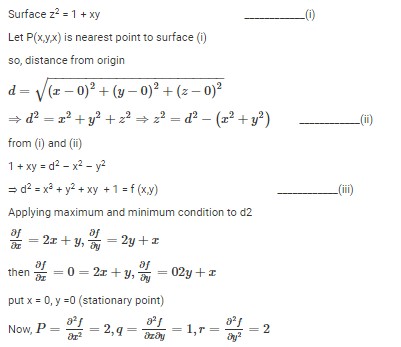Courses

# The distance between the origin and the point nearest to it on the surface z2 = 1 + xy is a)1b)√3/2c)√3d)-2Correct answer is option 'A'. Can you explain this answer? Related Test: Partial Derivatives, Gradient - MCQ Test 1

## Mechanical Engineering Question

By Vivek Jangir · 4 weeks ago ·Mechanical Engineering
Divya Gaur answered Jul 16, 2018or pr – q^2 = 4 – 1 = 3 > 0 and r = +ve

so f(xy) is minimum at (0,0)

Hence, minimum value of d^2 at (0,0)

d2 = x^2 + y^2 + xy + 1 = (0)^2 + (0)^2 + (0)(0) + 1 = 1

Then the nearest point is

z^2 = 1 + xy = 1+ (0)(0) = 1

or z = 1

Kunal Nayak answered 4 weeks ago
The answer is A because the co -ordinates of origin is zero and nearest point of the origin tends to zero so putting the values that's very small change which is negligible so answer is 1